Courses

## 20 Questions MCQ Test Mathematics (Maths) Class 8 | Test: Understanding Quadrilaterals- 2

Description
This mock test of Test: Understanding Quadrilaterals- 2 for Class 8 helps you for every Class 8 entrance exam. This contains 20 Multiple Choice Questions for Class 8 Test: Understanding Quadrilaterals- 2 (mcq) to study with solutions a complete question bank. The solved questions answers in this Test: Understanding Quadrilaterals- 2 quiz give you a good mix of easy questions and tough questions. Class 8 students definitely take this Test: Understanding Quadrilaterals- 2 exercise for a better result in the exam. You can find other Test: Understanding Quadrilaterals- 2 extra questions, long questions & short questions for Class 8 on EduRev as well by searching above.
QUESTION: 1

### Which of the following is true for the adjacent angles of a parallelogram?

Solution: Yes off course see 50+y =180 (co-interior angle) y =180-150 y =130
QUESTION: 2

Solution:
QUESTION: 3

### Which of the following quadilaterals has two pairs of adjacent sides equal and diagonals intersecting at right angles?

Solution:

Kite has two adjacent sides equal and since it’s a rhombus it’s diagonals bisect each other at right angles

QUESTION: 4

RICE is a rhombus. Find x.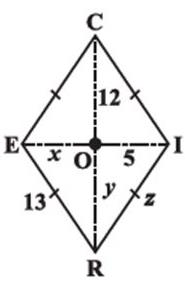Solution:

Given a rhombus RICE where
RE = 13, Ol = 5 OC = 12
In a rhombus,
Diagonals bisect each otherAlso,
All sides of a rhombus are equal.
RI = RE
x = 5
x = 5, y = 12 and z =13

QUESTION: 5

RICE is a rhombus. Find z.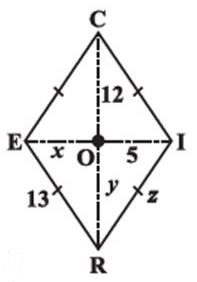Solution:

Given a rhombus RICE where
RE = 13, Ol = 5 OC = 12
In a rhombus,
Diagonals bisect each otherAlso,
All sides of a rhombus are equal.
RI = RE
z = 13
x = 5, y = 12 and z =13

QUESTION: 6

State the name of a regular polygon of 6 sides.

Solution:
QUESTION: 7

Find the measure of each exterior angle of a regular polygon of 9 sides.

Solution:
Sum of all exterior angles of the given polygon = 360degree
Each exterior angle of a regular polygon has the same measure.
Thus, measure of each exterior angle of a regular polygon of 9 sides=360/9= 40degree.
QUESTION: 8

The sides of a pentagon are produced in order. Which of the following is the sum of its exterior angles?

Solution:
QUESTION: 9

How many diagonals does a rectangle have?

Solution:
QUESTION: 10

ABCD is a quadrilateral. If AC and BD bisect each other, what is ABCD?

Solution: Sum of all angles of a quadrilateral is 360 degree . so we will add all the angles of quadrilateral and subtract it from 360 to get x. there is a linear pair on left side and exterior angle is 90 hence the other angle will be 90 by linear pair property. hence 70+60+90+x=360 220+x=360 x=360-220 x=140 hence C is the right answer.
QUESTION: 11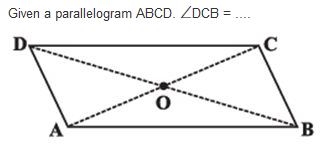Solution:
QUESTION: 12

A _______ is both ‘equiangular’ and ‘equilateral’.

Solution: A regular polygon is eqiangular and equilateral as even the sides of such a polygon are equal. To verify u can use properties of a polygon(quadrilateral is the easiest).
QUESTION: 13

A _________ has all the properties of a parallelogram and also that of a kite.

Solution:
QUESTION: 14

Find the value of the unknown y.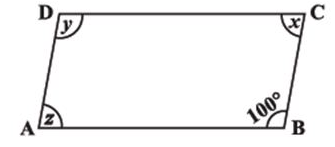Solution:
QUESTION: 15

A simple closed curve made up of only line segments is called a ________.

Solution:
QUESTION: 16

A _________ is a quadrilateral whose opposite sides are parallel.

Solution:
QUESTION: 17

Find the value of the unknown x.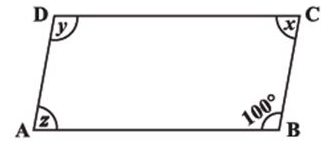Solution:

We have sum of adjacent angles equal to 180, so x+100=180x=80

QUESTION: 18

RENT is a rectangle. Its diagonals meet at O. Find x, if OR = 2x + 4 and OT = 3x + 1.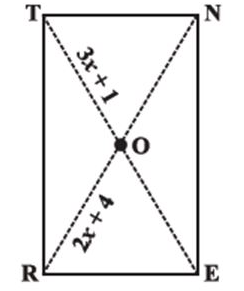Solution:

2x+4 = 3x+1 gives x = 3

QUESTION: 19

How many diagonals does a triangle have?

Solution:

A triangle has zero diagonals.Diagonals must be created across vertices in a polygon, but the vertices must not be adjacent to one another. A triangle has only adjacent vertices.

QUESTION: 20

Find the angle measure x in the following figure: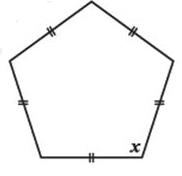Solution:

We have angle sum of pentagon=(5-2)*180 =540. Since it is a regular pentagon all angles are equal. So the sum is 5x. So each angle measures  x = 540/5 = 108º